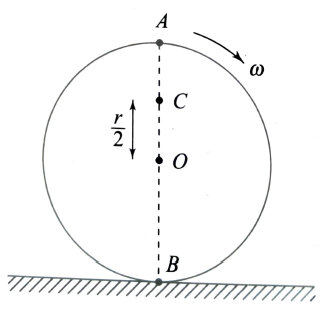Rotational motion
Question

# A disc of radius r = 20 cm is rotating about its axis with an angular speed of 20 rad s-1. It is gently placed on a horizontal surface which is perfectly frictionless (Fig). What is the linear speed of point A on the disc (in m/s)?Moderate
Solution

## Since the surface is perfectly frictionless, the disc will not roll on the surface; it will simply keep on rotating at point B where it is placed. NowLinear speed = distance from centre $×$ angular speedGiven, $\therefore$Linear speed at point

Get Instant Solutions